# Non-Cartesian MR Image Reconstruction Without Dependencies

Mark Chiew (mark.chiew@ndcn.ox.ac.uk)
In this tutorial, I will demonstrate how to perform iterative, non-Cartesian MR image reconstruction in MATLAB with no dependencies on external toolboxes or libraries. While excellent resources such as Jeff Fessler's NUFFT toolbox exist, my goal here is to lay everything out as explicitly as possible as a learning tool, relying on very little prior knowledge, and using only vanilla MATLAB.
I will present a worked example, reconstructing a test image from a simulated variable-density spiral k-space trajectory by solving both L2-regularised and compressed sensing (using total variation) problems, using a simple gradient descent algorithm.
The code used to generate this tutorial is available to download:
NB: The choices made here were for clarity and ease of understanding, and do not relect best practices for real reconstruction problems.
Warning: Adding local functions to scripts is only supported from R2016b onwards. If using an older version of MATLAB, move the function definitions to separate files, or wrap the main script inside of its own function. I've also only really tested this live script interface on R2018a, and it may not look quite the same on older versions of MATLAB. The plain script should run fine, however.

## Define our test object

N_x = 64; % size of image
x = phantom(N_x); % initialise phantom image
% show our source image
figure();imshow(x,[0 1]); title('Source Image');drawnow;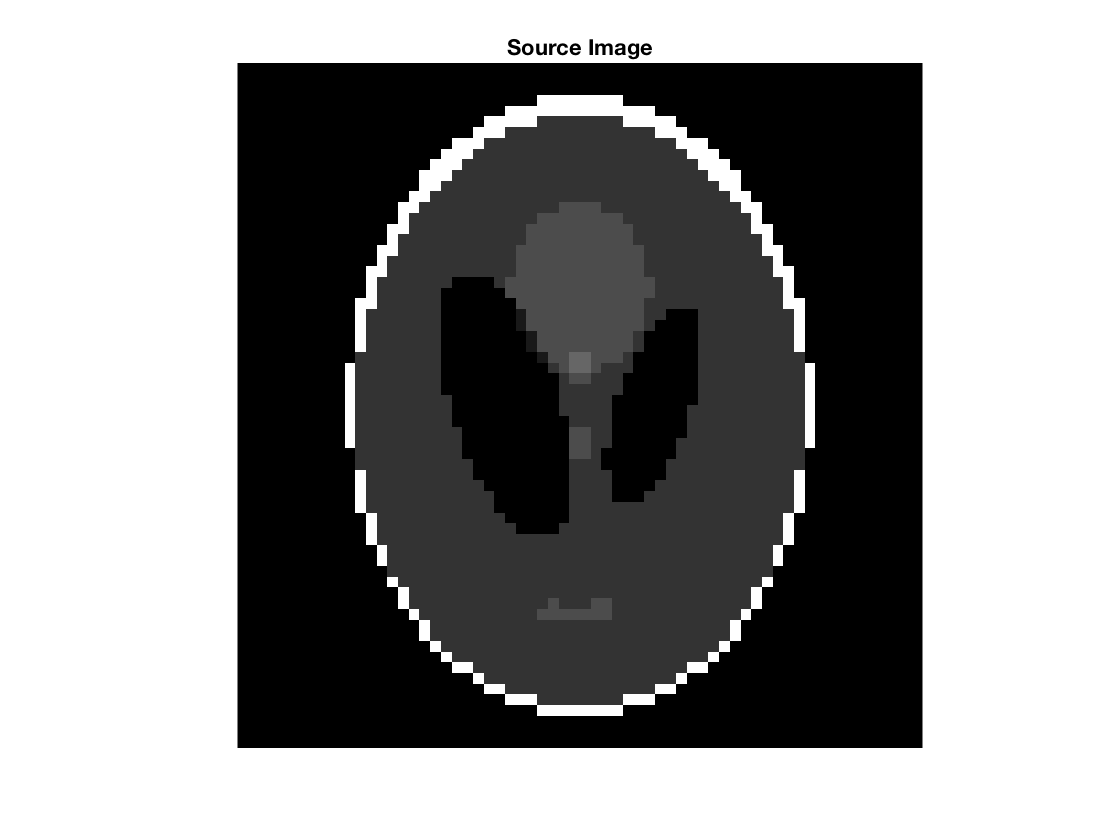## Define arbitrary k-space sampling locations

Our 2D non-Cartesian k-space samples should be defined over a 2D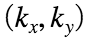plane, where the number and location of samples is defined by your k-space readout trajectory. In this case, we simulate (in an entirely unrealistic way) a randomly perturbed variable density spiral. However, any trajectory can be described simply as an array ofsamples.
If we assume our image space to be defined by voxel units (i.e.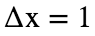in voxel units), thenandmeans that yourshould be scaled between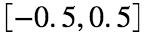.
% Perturbed variable density spiral
N_k = 64^2; % number of k-samples
t = linspace(0,sqrt(0.5),N_k)'; % dummy variable to parameterise spiral
k_x = (1 + randn(N_k,1)/20).*t.^2.*cos(2*pi*32*t); % spiral kx-coords
k_y = (1 + randn(N_k,1)/20).*t.^2.*sin(2*pi*32*t); % spiral ky-coords
% show scatterplot of trajectory
figure();scatter(k_x,k_y,5,'filled');axis square;grid on
xlabel('k_x');ylabel('k_y');title('k-space Sampling Locations');drawnow;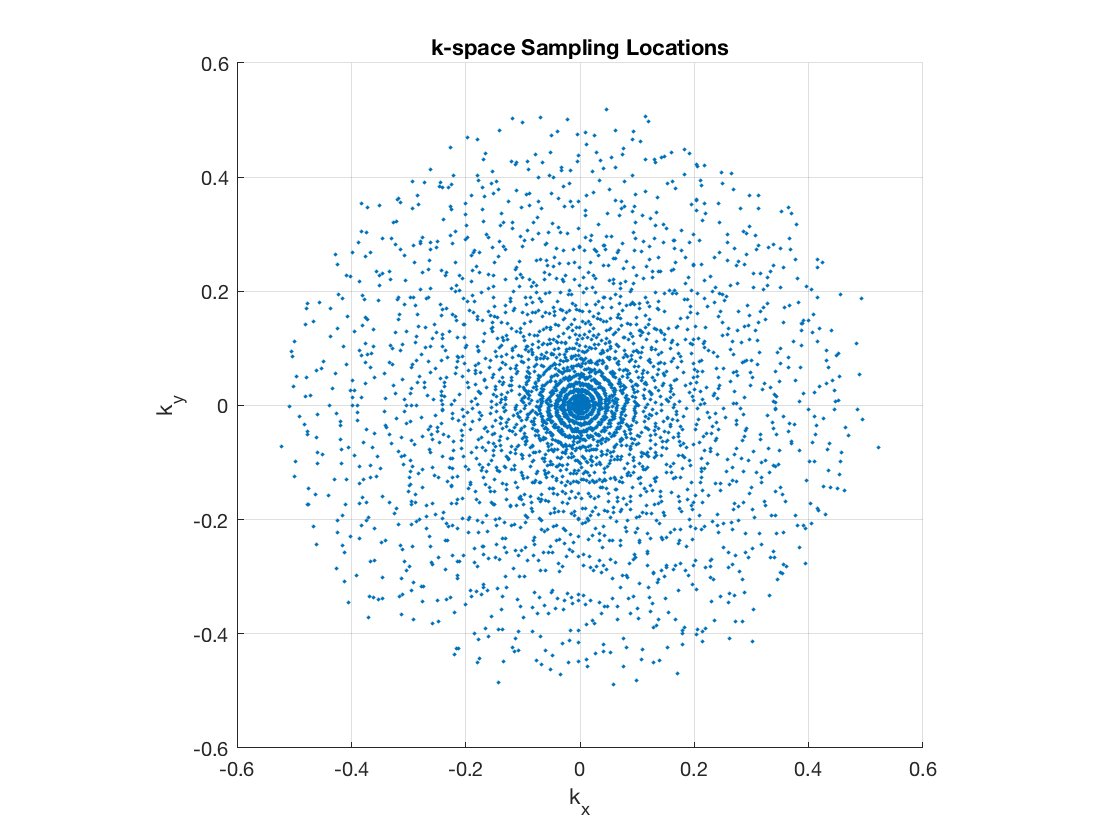## Define discrete Fourier encoding matrix

Here we explicitly construct our "forward model" for MR data acquisition: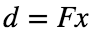,
where d is the measured k-space data, F is our measurement operator, and x is our object/image.
The data at k-space location corresponds to the sum over all space of the image multiplied with a 2D complex exponential with spatial frequencies k_x and k_y in the x- and y-directions respectively. This describes a linear function (no surprise, as the Fourier transform is linear), and we can represent it in the discrete case as an encoding matrix F. Each row of the encoding matrix represents the measurement at a single k-space location.
I'll note here that our forward model is discrete, but in reality, the forward encoding of MR data is essentially a continuous process. This choice reflects certain assumptions about our voxel basis functions, which we won't go into detail here.
[xidx, yidx] = meshgrid(-N_x/2 : N_x/2 - 1); % x- and y-coords with "isocentre" at the N_x/2+1 index
% Loop over each k-location to construct its required spatial phase modulation
F = zeros(N_k, N_x, N_x);
for i = 1:N_k
F(i,:,:) = exp(1j*2*pi*(k_x(i)*xidx+k_y(i)*yidx)); % 2D Fourier exponential
end
F = reshape(F, N_k, []); % reshape F so that each row is a single k-space encoding
% show an example encoding
figure();imshow(angle(reshape(F(2000,:),N_x,N_x)),[-pi pi],'colormap',hsv);
title('Phase of Example 2D Fourier Encoding Modulation');colorbar();drawnow;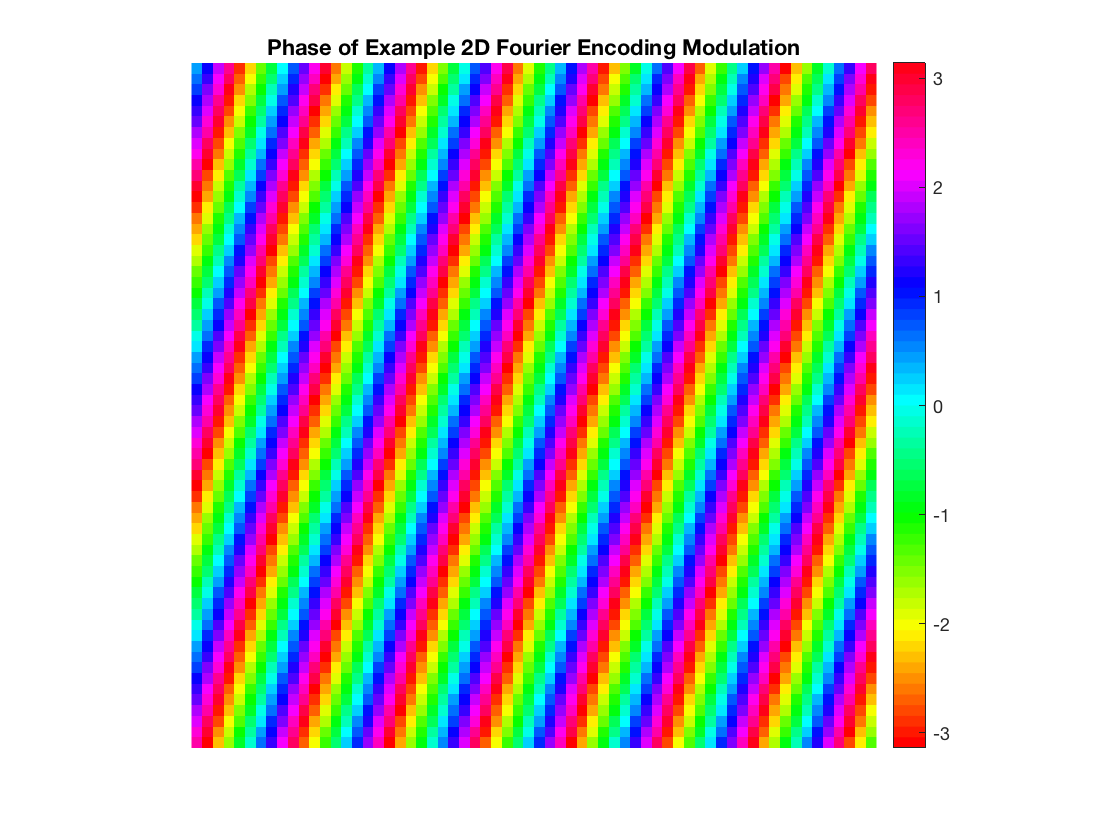## Perform forward encoding/measurement

We simulate data acquisition by running our image through the forward model. We first vectorize our image (using the same column-by-column MATLAB default reshaping as done for the encoding matrix F), then simply perform a matrix-vector multplication.
In reality, we start our image reconstruction problems here, with d provided by the scanner, and the job of image reconstruction to find the image that corresponds "best" to the measured data in some sense. That is, we solve the inverse problem of finding x given d and F.
d = F*x(:); % multiply encoding matrix with image vector to get data

## Direct Inverse Reconstruction?

A direct inverse is often impractical or impossible in non-Cartesian imaging because:
• F is often never explicitly constructed
• F has no inverse unless it is square (same number of k-space points and voxels) and non-singular
• when F is square and non-singular, it can still be very poorly conditioned
• when F is large enough, explicit inversion is slow and computationally intensive
In this example, F was constructed to be square to illustrate to examine the impact of system conditioning.
fprintf(1,'Condition number: %3.2G\n',cond(F)); % condition number of F
Condition number: 1.3E+20
est0 = F\d; % compute a naive inverse (warning: slow)
% print nrmse to the ground truth and show image and difference image
nrmse(est0, x, 'Direct Inverse'),plt(est0, x, N_x, 'Direct Inverse Est.');
Direct Inverse NRMSE: 436.117988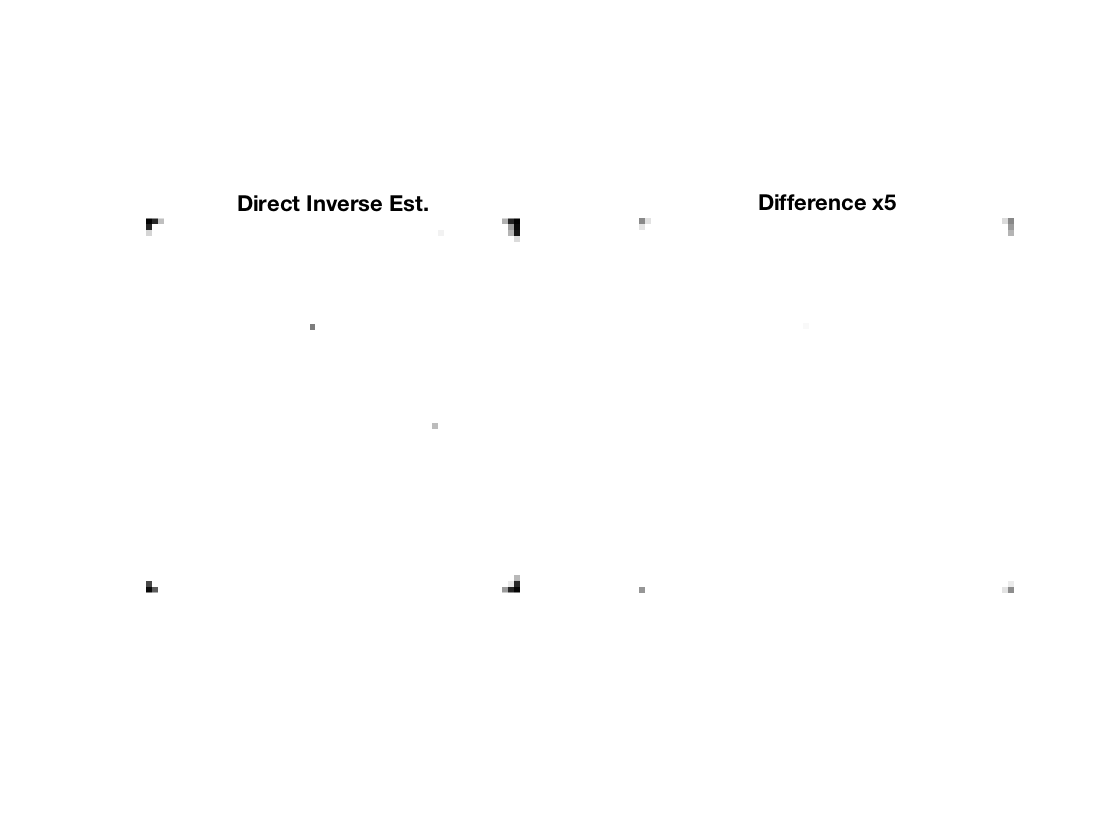## Direct Regularised Least Squares

To improve conditioning, and to accommodate the general case where the inverse problem is ill-posed (more or fewer k-space samples than voxels), we now choose to "solve" for x in the least-squares sense, with an additional L2-regularisation factor if desired/necessary. The extra term can help identify a unique solution and prevent over-fitting.
We want to minimize for x,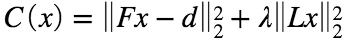where L is some other linear operator or transform, and λ is a relative weighting parameter between the data consistency least squares (first term) and L2-regulariser (second term).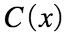is often referred to as the cost function. We solve for x by finding the value of x that minimises the cost. Note that the optimal value of x depends on how you define your cost (i.e. what regularisation is used, at what weighting), so what you get out depends entirely on what you ask for.
To directly solve this, we simply take the gradient (i.e. multivariate derivative) ofand set it to zero to find the value of x that optimises it. We take the gradient because our image is multivariate, where each voxel (or index in x) corresponds to its own variable.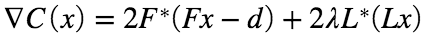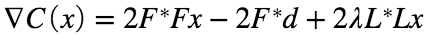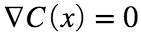means that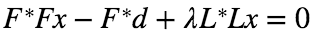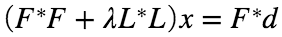Alternatively, rewrite the equation as, and so,means thatso the optimum is found at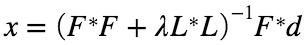For L2 regularisation penalties, the optimal estimator is linear (i.e., it doesn't depend on x directly). Furthermore, when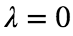(no regularisation), this reduces to the simple pseudoinverse. Note that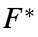denotes the adjoint (or conjugate transpose in discrete cases) of F.
Here we choose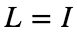, the identity matrix, which effectively penalises the energy of x.
lambda = 1E-4; % regularisation weighting
E = (F'*F+lambda*eye(N_x^2))\F'; % compute linear estimator (warning: slow)
fprintf(1,'Condition number: %3.2G\n',cond(E)); % condition number of E
Condition number: 5.6E+14
est1 = E*d; % regularised reconstruction
% print nrmse to the ground truth and show image and difference image
nrmse(est1, x, 'Regularised Inverse'),plt(est1, x, N_x, 'Regularised Inverse');
Regularised Inverse NRMSE: 0.338165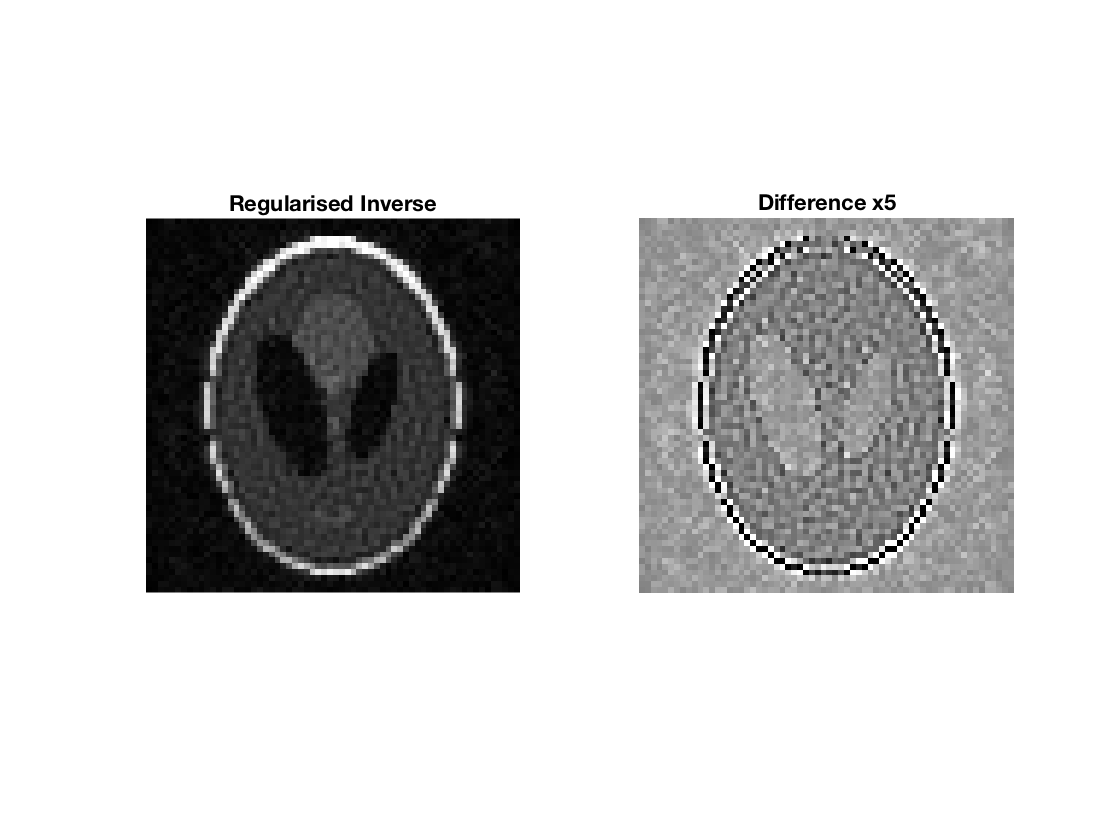## Iterative Regularised Least Squares

Because direct inversion is still slow and costly, we can solve the same cost function with no explicit inverse operations, by using iterative schemes. Lets consider the simplest case of a steepest gradient descent algorithm: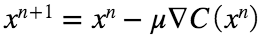where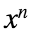is the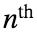estimate of x, μ is a step size, and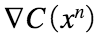is the gradient of the cost evaluated at.
We can also simplify the gradient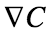as: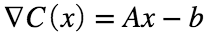where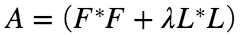and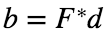.
While it does seem like extra work, these procedures, and other first order (gradient only) iterative methods like it (e.g. conjugate gradient methods) are often much more computationally efficient, particularly when the dimensionality of x gets large.
Here, we continue using, and we define a small fixed step size for simplicity (although you can estimate a more efficient, adaptive step size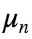at every iteration). We define a maxinum number of iterations, but will stop iterating if the relative change in our estimateis small enough.
% simplifying substitutions
A = F'*F + lambda*eye(N_x^2);
b = F'*d;
% define the gradient as a function, grad(x) = gradient(C(x))
grad = @(x) A*x - b;
% define a fixed step size, max number of iterations, and relative change tolerance
step = 1E-6;
iter = 1000;
tol = 1E-6;
% define an initial guess (starting point)
est2 = zeros(N_x^2,1);
% gradient descent function defined at the bottom
% ===================================================================
%{
function x = grad_desc(x, grad, step, max_iter, tol)
% steepest gradient descent
ii = 0; % iteration counter
dx = inf; % relative change measure
while ii < max_iter && dx > tol % check loop exit conditions
tmp = step*grad(x); % compute gradient step
dx = norm(tmp)/norm(x); % compute relative change metric
x = x - tmp; % update estimate
ii = ii+1; % update iteration count
end
end
%}
% ===================================================================
% run through steepest gradient descent
est2 = grad_desc(est2, grad, step, iter, tol);
% print nrmse to the ground truth and show image and difference image
nrmse(est2, x, 'Iterative L2 Reg'),plt(est2, x, N_x, 'Iterative L2 Reg');
Iterative L2 Reg NRMSE: 0.395185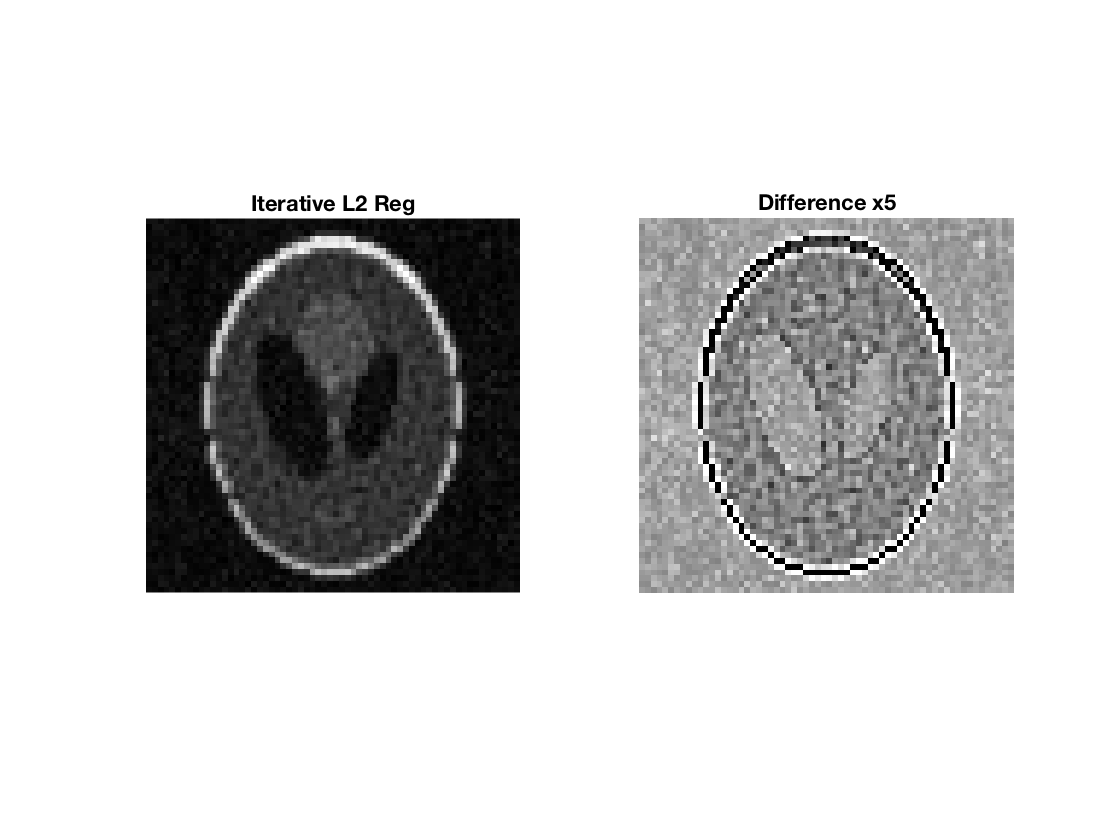## Using built-in linear solvers for L2 penalties

Becauseamounts to solving another (conjugate) symmetric linear system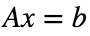, we can also use any of MATLAB's builtin symmetric linear solvers (e.g. pcg, minres):
% using pcg
est3 = pcg(A,b,tol,iter);
pcg converged at iteration 240 to a solution with relative residual 9.6e-07.
% print nrmse to the ground truth and show image and difference image
nrmse(est3, x, 'pcg'),plt(est3, x, N_x, 'pcg estimate');
pcg NRMSE: 0.360801